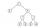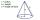# Compare:

What is bigger?
« Correct result#### Solution:Leave us a comment of example and its solution (i.e. if it is still somewhat unclear...):

Showing 0 comments:Be the first to comment!## Next similar examples:

1. Dropped sheetsThree consecutive sheets dropped from the book. The sum of the numbers on the pages of the dropped sheets is 273. What number has the last page of the dropped sheets?
2. Negative in equation2x + 3 + 7x = – 24, what is the value of x?
3. WithdrawalIf I withdrew 2/5 of my total savings and spent 7/10 of that amount. What fraction do I have in left in my savings?
4. ExpressionsFind out value of expressions if a = -1, b =2: x=b - 2a - ab y=a3 - b2 - 2ab z=a2 b3 - a3 b2 w=a + b + a3 - b2
5. RoundingThe following numbers round to the thousandth:
6. Round it0.728 round to units, tenths, hundredths.
7. HotelThe hotel has a p floors each floor has i rooms from which the third are single and the others are double. Represents the number of beds in hotel.
8. Find xSolve: if 2(x-1)=14, then x= (solve an equation with one unknown)
9. Write 2Write 791 thousandths as fraction in expanded form.
10. AlleyAlley measured a meters. At the beginning and end are planted poplar. How many we must plant poplars to get the distance between the poplars 15 meters?
11. TeacherTeacher Rem bought 360 pieces of cupcakes for the outreach program of their school. 5/9 of the cupcakes were chocolate flavor and 1/4 wete pandan flavor and the rest were vanilla flavor. How much more chocolate flavor cupcakes than vanilla flavor?
12. Equation 29Solve next equation: 2 ( 2x + 3 ) = 8 ( 1 - x) -5 ( x -2 )
13. The diagramThe diagram is a cone of radius 8cm and height 10cm. The diameter of the base is. ..
14. Cupcakes 2Susi has 25 cupcakes. She gives 4/5. How much does she have left?
15. NormThree workers planted 3555 seedlings of tomatoes in one dey. First worked at the standard norm, the second planted 120 seedlings more and the third 135 seedlings more than the first worker. How many seedlings were standard norm?
16. Simple equationSolve the following simple equation: 2. (4x + 3) = 2-5. (1-x)
17. DecideThe rectangle is divided into seven fields. On each box is to write just one of the numbers 1, 2 and 3. Mirek argue that it can be done so that the sum of the two numbers written next to each other was always different. Zuzana (Susan) instead argue that.